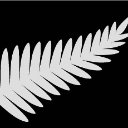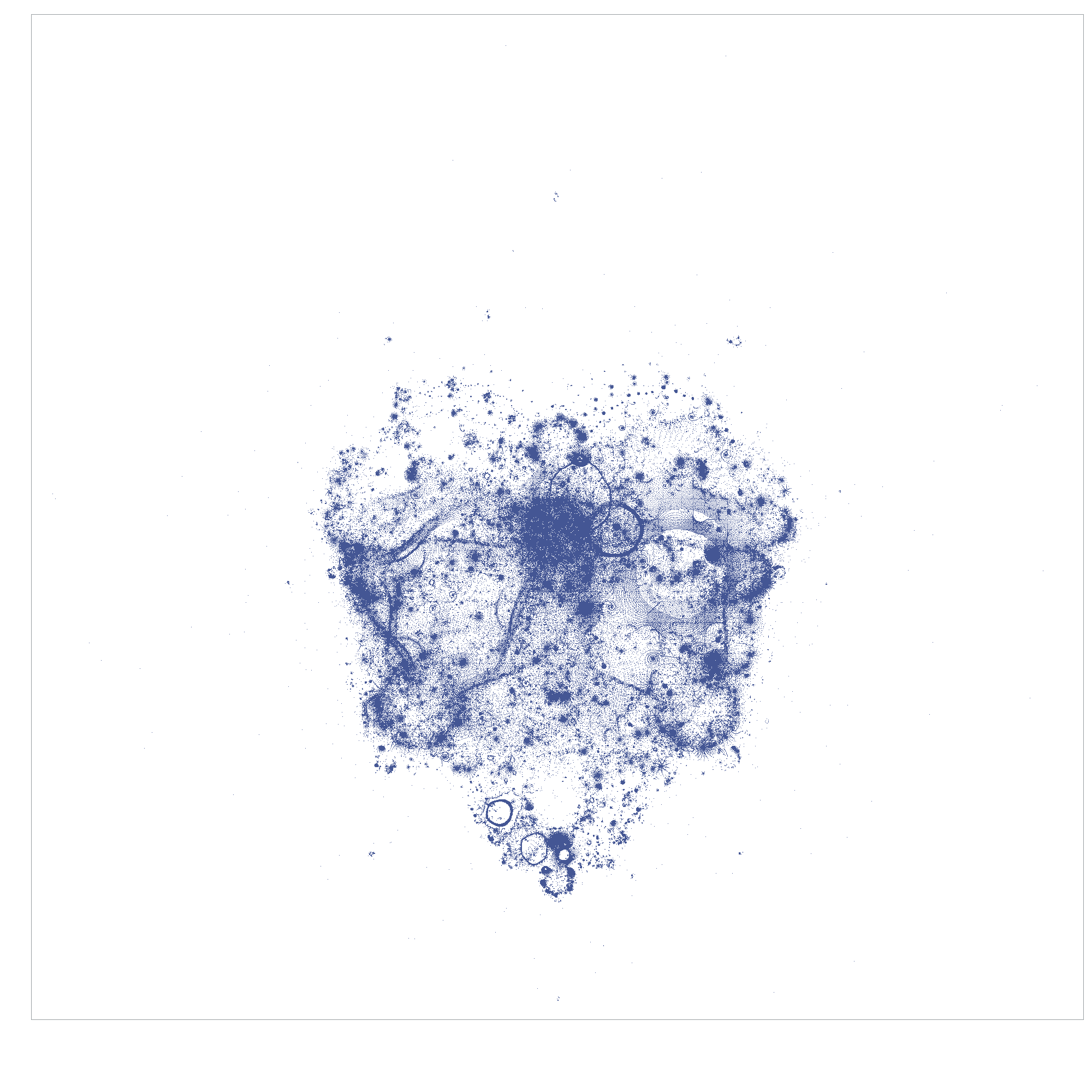ChrisNZ
Tourmaline | Level 20

## I want the graphing area to be exactly 2000 pixels

I want to display pixels at exact locations, but I end up with a badly sized image file.

My graphic is exactly 2000 pixels wide, so I ask SAS to create a graphics output area of 2000x2000, but the parameters are changed by SAS. The image below should be 2096x2096 pixels in total, with the graphics output area 2000x2000 pixels and 96 pixels for the margins. However SAS decides to save the image itself as 2000x2000, and so the graphics output area is too small.Here is the code. Don't pay attention to the data, it is still work in progress and has issues. I only give it to reproduce the issue.

The graphing part is at the end.

``````/*data _null_;  %macro loop; %do i=1 %to 500000; a=2.2*2.2;%end; %mend;%loop;run;*/
/*data _null_;  %macro loop; %do i=1 %to 500000; a=2.2**2 ;%end; %mend;%loop;run;*/

%let NbPixels        =  2000;                      %* pixel definition of image produced ;
%let NbMaxIterations = 10000;                      %* maximum nb of iterations ;

data ITERATIONS;
array ZR (&NbIterations)           _temporary_ ; %* list of the real values of the visited points during an iteration;
array ZI (&NbIterations)           _temporary_ ; %* list of the imaginary values of the visited points during an iteration;
array COUNTS (&NbPixels,&NbPixels) _temporary_ ; %* list of the X,Y pixel values when an iteration succeeds;
array XPOINT (&NbPixels)  ;                      %* list of pixel X values used to write data set one row at a time;
NbMinIterations    =  1000;                      %* Discard points that take less than this many iterations to diverge ;
NbMaxIterations    = &NbMaxIterations;           %* Discard points that take more than this many iterations to diverge ;
NbPointsToPlot     =  2000;                      %* Number of random points that we want to plot    ;

do while (NbPointsComputed < NbPointsToPlot);                    %* Loop to find a point that will diverge after ;
%*    the required number of iterations;
call missing( of ZR[*], of ZI[*] );                            %* Reset list of points visited by iterations;

do until                                                       %* Find a starting point that ensure it isn´t diverging ;
(not( ZR*ZR + ZI*ZI  > 4                  %* point is outside of large circle ;
| P - 2*P*P + 0.25           > ZR              %* point is inside large cardiod    ;
| (ZR+1)*(ZR+1) + ZI*ZI < 1/16  ));   %* point is inside the circle       ;

ZR = 4*(ranuni(0)-0.5) ;                                  %* real part of point between -2 and +2 ;
ZI = 4*(ranuni(0)-0.5) ;                                  %* imaginary part of point between -2 and +2 ;
P = sqrt((ZR - 0.25)*(ZR - 0.25) + ZI*ZI);       %* calculate if the point is within main cardiod;
end  ;

do I = 1 to NbMaxIterations-1 ;                                %* point will be iterated to see if it diverges ;
newZI = 2*ZI[I]*ZR[I] + ZI;                               %* iterate the real part of the point ;
newZR = ZR[I]*ZR[I] - ZI[I]*ZI[I] + ZR;                   %* iterate the imaginary part of the point ;
if (newZR*newZR + newZI*newZI) < 4 then do;                  %* the point isn´t diverging, keep its values;
ZI[I+1] = newZI;
ZR[I+1] = NewZR;
end;
else leave;                                                  %* the point is diverging, don´t add it and stop iterating ;
end;

NbIterations=NbMaxIterations-nmiss(of ZR[*]);                  %* Number of iterations before divergence started;

if NbMinIterations <= NbIterations <= NbMaxIterations then do; %* if the point diverged within the chosen range, keep it;
do I = 1 to NbIterations;
xPos = round(0.5+0.25*&NbPixels.*(ZR[I] + 2));
yPos = round(0.5+0.25*&NbPixels.*(ZI[I] + 2));
COUNTS(xPos, yPos) + 1;
end ;
NbPointsComputed + 1;                                        %* Count the number of points found;
end ;

if NbPointsComputed*100/NbPointsToPlot > PercentComplete then do; %* Display how much of the desired computation has been done;
PercentComplete + 1;
putlog PercentComplete  '% completed.';
end ;
end  ;
do I= 1 to &NbPixels;
do J= 1 to &NbPixels;
XPOINT[J]=COUNTS[I,J];
end;
output;
end;
keep XPOINT:;
run;

data PLOT;
set ITERATIONS;
array XPOINT (&NbPixels)  ;
Y=_N_;
do X= 1 to &NbPixels;
VAL=XPOINT[X];
if VAL then output;
end;
keep X Y VAL;
run;

goptions reset=all dev=png;
%* Device is 96 DPI. We add 0.5in margins around graphics output area;
goptions xmax=%sysevalf(&NbPixels./96+1)in  hsize=%sysevalf(&NbPixels./96)in  xpixels=%eval(&NbPixels.+96);
goptions ymax=%sysevalf(&NbPixels./96+1)in  vsize=%sysevalf(&NbPixels./96)in  ypixels=%eval(&NbPixels.+96);

symbol h=0.1 v=dot;
proc gplot;
plot Y*X /noaxis nolegend;
run;
quit;
``````

1 ACCEPTED SOLUTION

Accepted SolutionsChrisNZ
Tourmaline | Level 20

## Re: I want the graphing area to be exactly 2000 pixels

Trial and error seems to be the only method to set the size of the graphics output area, and the documentation is misleading at best.

Not good! 😞

Using these values yields what I need:

goptions reset=all dev=png xpixels= 548 ypixels= 584 ; * 500x500 ;

goptions reset=all dev=png xpixels=2094 ypixels=2215 ; * 2000x2000 ;

symbol v=dot h=0.01;

data T;

do I=1 to 2000;

X=I; Y=1 ;output; Y=2000 ;output;

Y=I; X=1 ;output; X=2000 ;output;

end;

run;

proc gplot;

plot Y*X / noaxis nolegend;

run;ChrisNZ
Tourmaline | Level 20

## Re: I want the graphing area to be exactly 2000 pixels

Trial and error seems to be the only method to set the size of the graphics output area, and the documentation is misleading at best.

Not good! 😞

Using these values yields what I need:

goptions reset=all dev=png xpixels= 548 ypixels= 584 ; * 500x500 ;

goptions reset=all dev=png xpixels=2094 ypixels=2215 ; * 2000x2000 ;

symbol v=dot h=0.01;

data T;

do I=1 to 2000;

X=I; Y=1 ;output; Y=2000 ;output;

Y=I; X=1 ;output; X=2000 ;output;

end;

run;

proc gplot;

plot Y*X / noaxis nolegend;

run;

Discussion stats